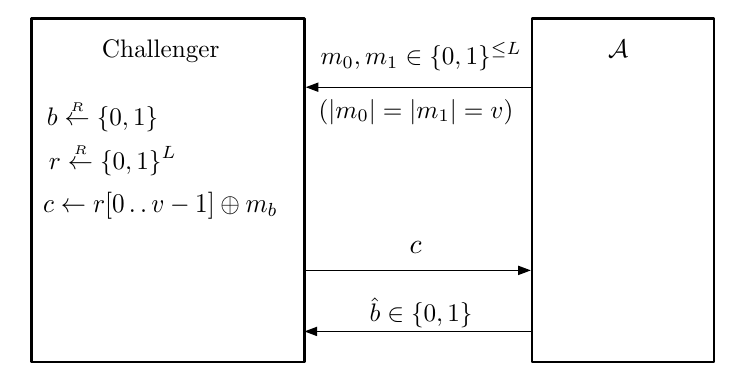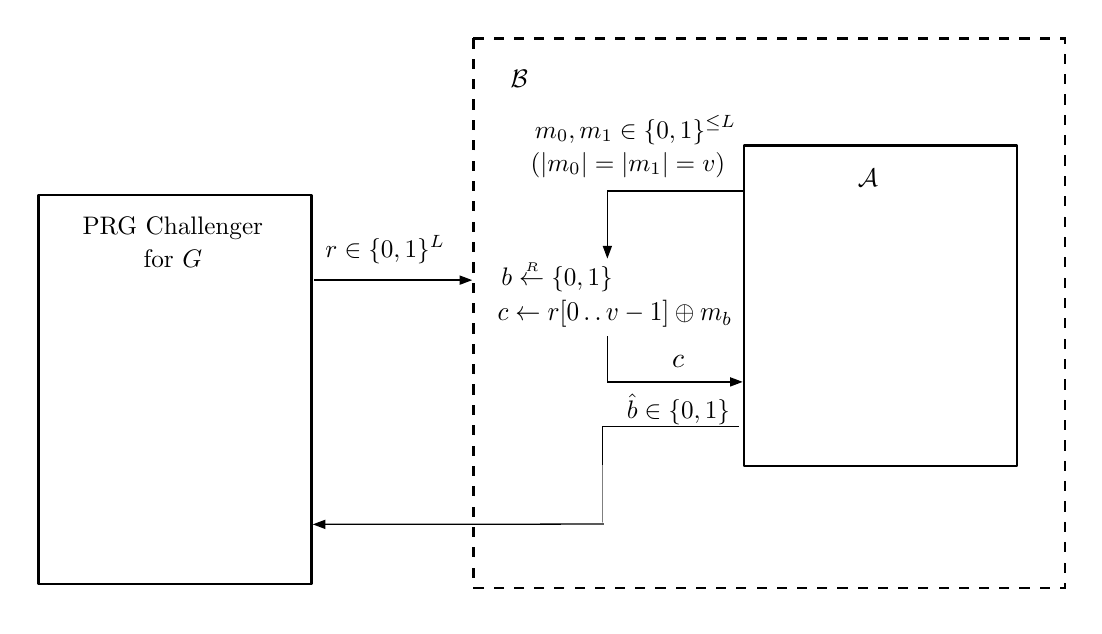All info comes from David Wu’s Lecture and Boneh-Shoup Book.

This note will be focusing mainly on perfect security, semantics security and PRG (Pseudo Random Generator).

The overall goal of cryptography is to secure communication over untrusted network. Two things must be achieved:

• Confidentiality: No one can eavesdrop the communication
• Integrity: No one can tamper with communication

# Perfect Security

A cipher $(Enc, Dec)$ satisfies perfect secure if $\forall m_0, m_1 \in M$ and $\forall c\in C$, $\Pr[k\overset{R}{\longleftarrow} K: Enc(k, m_0) = c] = \Pr[k\overset{R}{\longleftarrow} K:Enc(k,m_1) = c]$.

$k$ in two $\Pr$ might mean different $k$, the $\Pr$ just indicate the possibility of $\dfrac{\text{number of }k\text{ that }Enc(k, m) = c}{|K|}$.

## OTP is Perfect Secure

For every fixed $m = \lbrace 0, 1\rbrace^n$ there is $k, c = \lbrace 0, 1\rbrace^n$ uniquely paired that $m \oplus k = c$.

Considering perfect security definition, only one $k$ can encrypt $m$ to $c$. Thus $\Pr = \dfrac{1}{|K|} = \dfrac{1}{2^n}$ and equation is satisfied.

If a cipher is perfect secure, then $|K| \ge |M|$.

Assume $|K| < |M|$, we want to show it is not perfect secure. Let $k_0 \in K$ and $m_0 \in M$, then $c \leftarrow Enc(k_0, m_0)$. Let $S = \lbrace Dec(k, c): k \in K\rbrace$, we can see $|S| \le |K| < |M|$.

We can see that $\Pr\lbrack k \overset{R}{\longleftarrow} K: Enc(k, m_0) = c\rbrack > 0$, if we choose $m_1 \in M \backslash S$, then $\not\exists k \in K: Enc(k, m_1) = c$. Thus it is not perfect secure. $\square$

We can observe that perfect security requires a large key space, in both $|K|$ large or long $k$ string.

Given infinity computation power, adversary cannot break security, since $|K| = |C| = |M|$, and no information about $m$ can be retrieved by $c$ simply.

A even more generalized version is that, given an arbitrary $c$, $m_0, m_1\in M$, since the possibility to encrypt $m$ to a fixed $c$ is the same for $k \in K$, computation power does not help.

A large cost is required since we have to give the $k$ in advance, where $k$ might be huge.

# Semantic Security

We relaxed our security requirement on Shannon Theorem to get more flexibility on $k$ length. We only consider computationally feasible adversary here, rather than adversary with infinity computability.

## Predicate

We define a boolean function $\phi$ that takes $c$ and returns a boolean value.

It is easy to see that if cipher $\mathcal{E} = (E,D)$ is Perfect Security if and only if $\forall \phi,\Pr\lbrack k\overset{R}{\longleftarrow}K:\phi(\text{Enc}(k,m_0))\rbrack = \Pr\lbrack k\overset{R}{\longleftarrow}K:\phi(\text{Enc}(k,m_1))\rbrack$.

If $\mathcal{E} = (E,D)$ is perfectly secure, then let $S = \lbrace c \in C:\phi(c)\rbrace$ and we have
$$\Pr\lbrack k\overset{R}{\longleftarrow}K:\phi(\text{Enc}(k,m_0))\rbrack = \sum\limits_{c\in S} \Pr\lbrack k\overset{R}{\longleftarrow}K: \text{Enc}(k,m_0) = c \rbrack = \sum\limits_{c\in S} \Pr\lbrack k\overset{R}{\longleftarrow}K: \text{Enc}(k,m_1) = c \rbrack = \Pr\lbrack k\overset{R}{\longleftarrow}K:\phi(\text{Enc}(k,m_1))\rbrack$$
If $\Pr\lbrack k\overset{R}{\longleftarrow}K:\phi(\text{Enc}(k,m_0))\rbrack = \Pr\lbrack k\overset{R}{\longleftarrow}K:\phi(\text{Enc}(k,m_1))\rbrack$, suppose $\mathcal{E}$ is not perfect secure, we can construct a $\phi$ is only true for $c$.

Then $\Pr[k\overset{R}{\longleftarrow} K: Enc(k, m_0) = c] \neq \Pr[k\overset{R}{\longleftarrow} K:Enc(k,m_1) = c]$ is contradicted.

Semantic Security is a weaker definition than perfect security.

Consider a deterministic cipher $\mathcal{E} = (E, D)$ over $(K,M,C)$, we can relax perfect security $\Pr\lbrack k\overset{R}{\longleftarrow}K:\phi(\text{Enc}(k,m_0))\rbrack = \Pr\lbrack k\overset{R}{\longleftarrow}K:\phi(\text{Enc}(k,m_1))\rbrack$ to
$$|\Pr\lbrack k\overset{R}{\longleftarrow}K:\phi(\text{Enc}(k,m_0))\rbrack - \Pr\lbrack k\overset{R}{\longleftarrow}K:\phi(\text{Enc}(k,m_1))\rbrack| \le \epsilon$$

## Attack Game Experiment and Advantage

The attack game or experiment can help formulate semantic security. Two experiments are involved and indexed by $b \in \lbrace 0,1\rbrace$.

$\mathcal{A}$ may follow any efficient protocol, it chooses two messages and receives encryption of one of them, it needs to guess which one by returning $\hat{b}$ of which message.

The goal is to capture property that no efficient adversary can learn any information about the message given only then cipher text, or distinguish encrypted $m_0$ from encrypted $m_1$.Let $\begin{cases}W_0 = \Pr\lbrack\hat{b} = 1 | b=0 \rbrack\newline W_1 = \Pr\lbrack\hat{b} = 1 | b=1 \rbrack\end{cases}$ be probability that adversary guesses 1 in $b^\text{th}$ experiment.

If adversary knows something, then $W_0,W_1$ will be greatly different.

We can define the $\mathcal{A}$ adversary’s semantic security advantage w.r.t. $\mathcal{E}$ be $\text{SSadv}\lbrack \mathcal{A,E}\rbrack = |\Pr\lbrack W_0\rbrack - \Pr\lbrack W_1\rbrack|$.

If cipher $\mathcal{E}$ is semantically secure if $\forall \mathcal{A}, \text{SSadv}\lbrack \mathcal{A,E}\rbrack = |\Pr\lbrack W_0\rbrack - \Pr\lbrack W_1\rbrack| = \text{negl}(\lambda)$.

We can also prove “if $\mathcal{E}$ is perfectly secure, then it is semantically secure” in this perspective.

Since we can let
$$\underbrace{\Pr[k\overset{R}{\longleftarrow} K: Enc(k, m_0) = c]}_{W_0} = \underbrace{\Pr[k\overset{R}{\longleftarrow} K:Enc(k,m_1) = c]}_{W_1}$$
be two experiments, by previously discussed predicate function we cannot infer any information from $\phi$ on $m_0, m_1$ about $c$, thus $\text{SSAdv}\lbrack \mathcal{A,E}\rbrack = 0$.

# Pseudo Random Generator and Stream Cipher

The idea is to compress the OTP key, by generating a long random-looking string from a small seed. $s \in \lbrace 0, 1\rbrace^\lambda \to G(s) \in \lbrace 0,1\rbrace^n$, where $n \gg \lambda$ and $G$ s a deterministic algorithm.

This is PRG (Pseudo Random Generator), and the indistinguishable feature from OPT goes to computationally indistinguishable feature.

The security is discussed in the following attack game (experiment) just like semantic security.The $\mathcal{A}$ advantage towards $G$ this pseudo random generator is defined as $\text{PRGAdv}\lbrack\mathcal{A},G\rbrack = | W_0 - W_1 |$ where $\begin{cases}W_0 = \Pr\lbrack\hat{b} = 1 | b=0 \rbrack\newline W_1 = \Pr\lbrack\hat{b} = 1 | b=1 \rbrack\end{cases}$.

A PRG $G : \lbrace 0,1\rbrace^\lambda \to \lbrace 0,1\rbrace^n$ is secure if $\forall \text{efficient }\mathcal{A}$, $\text{PRGAdv}\lbrack\mathcal{A},G\rbrack = \text{negl}(\lambda)$.

Efficient here means probabilistic polynomial time.

## PRG Stream Cipher is Semantically Secure

We can formulate PRG OTP with following function.

$$k = \lbrace 0, 1\rbrace^\lambda, m=c=\lbrace0,1\rbrace^n, \begin{cases} \text{Enc}(k,m) & c \longleftarrow m \oplus G(k)\newline \text{Dec}(k, c) &m\longleftarrow c \oplus G(k)\end{cases}$$
Suppose $G$ is secure, then PRG OPT is semantically secure.

We can construct similar experiment that $b^\text{th}$ experiment chooses $m_0,m_1$ and receives $c_b = G(s)\oplus m_b$ where $s\overset{R}{\longleftarrow} S$. $\begin{cases}W_0 = \Pr\lbrack\hat{b} = 1 | b=0 \rbrack\newline W_1 = \Pr\lbrack\hat{b} = 1 | b=1 \rbrack\end{cases}$. We want to show $|W_0 - W_1| = \text{negl}(\lambda)$.

Since $|W_0 - W_1 | \le |W_0 -W_0’ | + |W_0’ - W_1’| + |W_1’ - W_1|$, we let $W’$ be experiment distinguishing PRG OPT and random key OPT. Since OPT is perfectly secure, $|W_0’ - W_1’| = 0$.

$W’$: $b^{\text{th}}$ experiment chooses $m_0,m_1$ and $c_b = m_b \oplus k$ where $k \overset{R}{\longleftarrow}\lbrace 0,1\rbrace^n$. $\begin{cases}W_0’ = \Pr\lbrack\hat{b} = 1 | b=0 \rbrack\newline W_1’ = \Pr\lbrack\hat{b} = 1 | b=1 \rbrack\end{cases}$.

We want to show if $G$ is secure for efficient $\mathcal{A}$, $|W_b - W_b’| = \text{negl}(\lambda)$ and we can prove the contrapositive.

Suppose there exists efficient $\mathcal{A}$ that can distinguish $W_b$ and $W_b’$, we want to prove $G$ is not secure. We construct attack game for $\mathcal{A}$.We can then construct $\mathcal{B}$ attack game on PRG distinguish with $\mathcal{A}$.On $\text{PRGAdv}\lbrack B,G\rbrack$, we can see $\begin{cases}\Pr\lbrack\hat{b} = 1 | b=0 \rbrack\newline \Pr\lbrack\hat{b} = 1 | b=1 \rbrack\end{cases}$ matches exactly with $W,W’$ experiment behavior, and thus $\text{PRGAdv}\lbrack B,G\rbrack = |W_0 - W_0’| = |W_1 - W_1’|$ and it is not negligible.

Since $B$ uses $\mathcal{A}$ as subroutine and $\mathcal{A}$ is efficient, $B$ is efficient.

$G$ is not secure. Thus contrapositive proved. $\square$